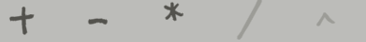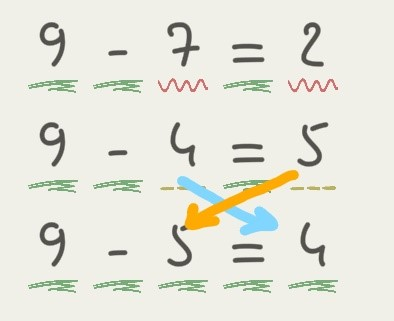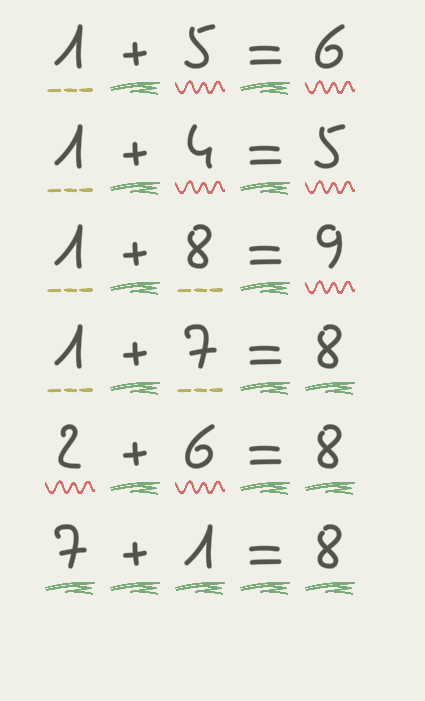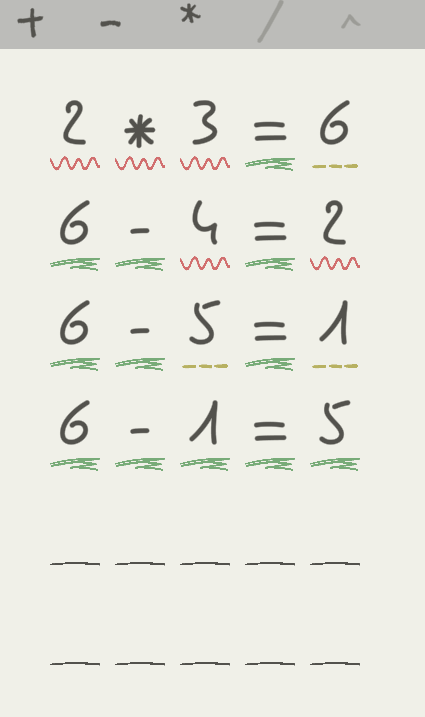# MATH EXPRESSions: Basic Guide (How to Find Each Expression)

This guide provides some guidance for how to play Math Expressions!

### Basic Introduction

Math Expressions is a game where you try to find out certain math expressions for each level. As of the time of typing this there are 5 spaces for numbers or arithmetic character operands (+,-,*,/,^). The base game only has these 5 arithmetic operands, addition, subtraction, multiplication, division and exponential. There are a few hints the game gives you to help guide you in the right direction, however these hints aren’t directly shown in game if you are blindly guessing expressions. In this guide I will be discussing methods that can be taken to find out each expression.

### How to play Math Expressions

First Lets Examine the basics of Math Expressions

As we open the game for the first time we can see that the only arithmetic operand is the plus sign (addition). Since this is the first expression we are requested to solve for, it’s safe to say that the plus will go in the second space for this round of expressions. The equals (“=”) sign will always go in the 4th spot within the 5 space expression space. Typically when we see a new arithmetic operand added to our above list (not grayed out anymore; see image below), that operand is to be used within the first expression.*This image shows the operand list with some unlocked and some locked*

For example: When the multiplication symbol appears on the next expression question, its safe to say we’re finding a product expression (expression with multiplication).

“Recognizing Hints from the game”

In order to help players find expressions its worth noting that there are three types of space underlines. A squiggle red, a dashed yellow, and a scribble green. A squiggle red underline means your input for that expression is wrong. A dashed yellow means the number you put in your expression is correct, but in the wrong place within the expression. A scribble green underline means that your number/ input is correct for that space.

Within the example below you can see, from the first line, the “9”, “-“, and “=” are in the correct space. However, the “7” & “2” are incorrect for this expression. I tried two different numbers in the next line. You can see they have the dashed yellow line beneath them, meaning they just need to be swapped in the expression for it to be correct. The third line shows our correct expression.Strategy

The basic strategy I’ve found is to guess your first expression based off of whether there is a new operand or not and pure guess. The next guess can be used to determine and correct a wrong sign. For these guesses (following the very first guess) try to spread out guesses on the spectrum of allowed numbers and use common sense.
For example you cant have more than 1 digit numbers in this case. Also I tend to find less success if you guess incrementally by 1 from your previous guess, instead of jumping from say 3 to 7. You may have better luck if you space out your numerical guesses.

In Summary

• Guess first based off context clues (for first guess, like if a new operand was added in)
• Use first guess and revise numbers or operands accordingly (all while keeping and eye out for the underlines)
• Use common sense (as mentioned)
• Switch things up if you have to
• Always have the “=” in the 4th space in the 5 space expressions

Hopefully this was of some help to some of you!!!

### Further Explanation (if you didn’t quite understand the steps)Explanation 1
For this expression you can see I have the 5 & 6 incorrect, and the 1 in the wrong spot. So within the next few steps I guessed until I found another number that was in the wrong spot, but in the expression otherwise. In guess 4, you can see I correctly relocated the 8 I guessed to the opposite side of the equals sign. In guess 5 you can see that I didn’t follow the previously listed method and tried to test something out (not relevant to this explanation). Then in the final guess you can see that I flipped the 7 & 1’s locations from guess 4. Something interesting worth noting here for addition expressions: In the world of mathematics 1+7=8 and 7+1=8 are correct either way because of the commutative law of addition.Explanation 2
Here you can see with my first guess 2,*, and 3 are incorrect and 6 is in the wrong spot. Since 6 is a larger number in our range from 0 to 9, I assumed it would go on the first spot of the expression, and a minus sign (since adding anything larger than 3 would give us a double digit number, which we cant have). In guess 3 you can see I guessed correctly in terms of 5 & 1, and only needed to swap their locations within the expression to get the correct answer.

By Haz